Talking about How to Implement the Design of Voltage Feedback Circuit in Current Mode Switching Power Supply

Abstract: In traditional voltage-type control, there is only one loop with poor dynamic performance. When the input voltage is disturbed, the duty cycle changes slowly through the voltage loop feedback. Therefore, in the case where the transient error of the output voltage is required to be small, the voltage type control mode is not preferable. In order to solve this problem, a current mode control mode can be employed. The design of the voltage feedback circuit in the current mode switching power supply will be described below.

1 circuit category, implementation of main function description

The circuit shown in the figure below belongs to the voltage feedback circuit. When the output voltage changes, the feedback circuit feeds back to the control chip to adjust the output voltage to stabilize the output voltage. The circuit is as follows: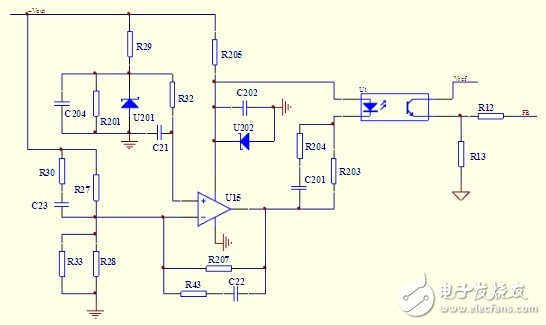Voltage feedback circuit

1.2 Analysis of working principle

When the output voltage changes, the voltage is divided by R27 and R28, and the voltage of the inverting input terminal of U15 changes. By comparing with the fixed voltage of the non-inverting input terminal of U15, the output voltage is amplified by the op amp, and the current through the photocoupler diode is passed. The change is transmitted to the output of the three-stage tube of the optocoupler, and then input to the control chip, and the control chip adjusts the output voltage to achieve stable output voltage.

2 uc3842 Introduction

Figure 1 shows the internal structure of the UC3842 PWM controller. The internal reference circuit generates a +5V reference voltage as the internal power supply of the UC3842, which is attenuated by 2.5V as the reference for the error amplifier, and can be used as a power supply for the circuit output 5V/50mA. The oscillator generates a square wave oscillation. The oscillation frequency depends on the external timing component. The resistor R connected between the 4 pin and the 8 pin and the capacitor C connected between the 4 pin and the ground determine the oscillation frequency of the oscillator, f= 1.8/RC. The feedback voltage is connected to the inverting terminal of the error amplifier by the 2-pin. The 1-pin external RC network is used to change the closed-loop gain and frequency characteristics of the error amplifier. The square-wave output of the 6-pin output drive switch is the totem pole output. The 3 pin is the current detection terminal, which is used to detect the current of the switch tube. When the voltage of the 3 pin is â‰¥1V, the UC3842 turns off the output pulse to protect the switch tube from overcurrent damage. The UC3842PWM controller features an undervoltage lockout circuit with a turn-on threshold of 16V and a turn-off threshold of 10V. This is why it can effectively prevent oscillations when the circuit is operating near the threshold voltage.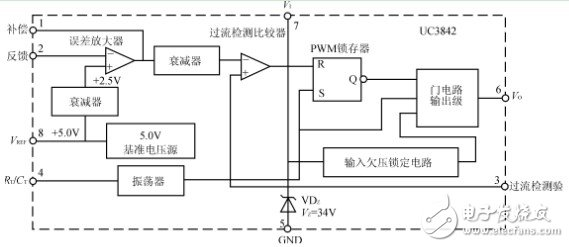1.1 UC3842 has the following features:

1. The number of pins is small, the peripheral circuit is simple, and the price is low;

2. The voltage adjustment rate is very good;

4, the frequency response characteristics are good, the stability is large;

5, with overcurrent limit, overvoltage protection and undervoltage lockout.

1.2 UC3842 has a good linear adjustment rate

Because the change of the input voltage Vi immediately reacts to the change of the inductor current, it can change the output pulse width in the comparator without any error amplifier, and then increase the output voltage of the first stage to the control of the error amplifier, which can make the linear adjustment rate more Good; the load regulation can be significantly improved because the error amplifier can be used to control the change of the output voltage due to the load change, especially the amplitude of the voltage rise under light load is greatly reduced. The external circuit compensation network of the error amplifier is simplified, the stability is improved and the frequency response is improved, and the gain bandwidth product is larger. The current limiting circuit is simplified. Since the peak inductor current is induced on the resistor, the pulse-by-pulse limiting circuit can be naturally formed. As long as the level of Rs reaches 1V, the PWM is immediately turned off, and the peak inductor current sensing technique can be sensitively limited. The maximum current output.

3 UC3842 commonly used voltage feedback circuit

3.1 Output voltage direct voltage division as the input of the error amplifier

The output voltage Vo is divided by two resistors and used as a sampling signal, and is input to UC3842 pin 2 (inverting input terminal of the error amplifier). As shown in Figure 2.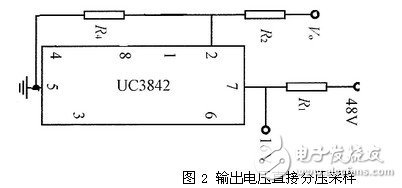The advantage of this circuit is that the sampling circuit is simple, and the disadvantage is that the input voltage and the output voltage must be common ground and cannot be electrically isolated. It is bound to cause difficulties in power supply wiring, and the power supply operates in a high-frequency switching state, which easily causes electromagnetic interference, which inevitably brings difficulties in circuit design, so this method is rarely used.

3.2 Auxiliary power supply output voltage divider as an input to the error amplifier

The induced voltage generated on the auxiliary winding of the single-ended flyback transformer T rises as the output voltage rises. This voltage is rectified, filtered, and stabilized to obtain a DC voltage to power the UC3842. At the same time, the voltage is divided by two resistors and used as the sampling voltage, and is sent to the foot of the UC3842.

When the UC3842 is started, if the feedback winding does not provide enough UF, the circuit will start continuously and snoring will occur. In addition, according to experience, if UF is greater than 17.5V, it will cause abnormal operation of UC3842, resulting in a smaller output pulse duty ratio and lower output voltage. Therefore, the selection of the feedback winding turns and its winding is very important. Generally, it can be designed according to 13~15V. When the UC3842 works normally, the voltage of the 7-pin is maintained at about 13V.

The advantage of this circuit is that the sampling circuit is simple, and there is no electrical path between the secondary winding, the primary winding and the auxiliary winding, and the wiring is easy. The disadvantage is that the sampling voltage is not directly obtained from the secondary winding, and the voltage stabilization effect is not good. It is found in the experiment that when the load of the power supply changes greatly, the voltage regulation cannot be basically achieved. This circuit is suitable for situations with certain fixed loads.

3.3 Using linear optocoupler to change the input error voltage of the error amplifier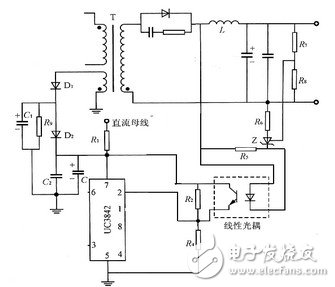As shown in Figure 3, the voltage sampling circuit of the switching power supply has two paths: First, the voltage of the auxiliary winding is rectified, filtered, and stabilized by D1, D2, C1, C2, C3, and R9 to obtain a DC voltage of 16V. UC3842 power supply, in addition, the voltage is divided by R2 and R4 to obtain a sampling voltage, the sampling voltage of the circuit mainly reflects the change of the DC bus voltage; the other is the optocoupler, the three-terminal adjustable voltage regulator Z and R4, R5, R6, R7, R8 voltage sampling circuit, the voltage reflects the change of the output voltage; when the output voltage rises, the reference voltage of the input Z is also increased after the voltage is divided by the resistors R7 and R8, the voltage regulator The regulation value increases, the current flowing through the LED in the optocoupler decreases, the current flowing through the phototransistor in the optocoupler decreases correspondingly, and the input feedback voltage of the error amplifier decreases, resulting in the output signal of the UC3842 pin 6 output. The duty cycle becomes smaller, and the output voltage drops, achieving the purpose of voltage regulation.

Because of the optocoupler, the circuit achieves isolation of output and input, isolation of weak current and strong electricity, reduces electromagnetic interference, and has strong anti-interference ability. It also samples the output voltage and has good voltage regulation performance. The disadvantage is that the number of external components increases, which increases the difficulty of wiring and increases the cost of the power supply.

3.4 Circuits with feedback control using optocouplers and voltage references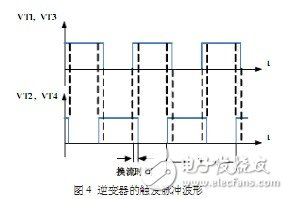In order to meet the power supply requirements when the load changes greatly. To improve the stability of the output voltage, a circuit for sampling feedback from the output of the secondary winding is designed. The circuit is shown in Figure 4. The voltage sampling and feedback circuit consists of optocouplers PC8I7, TL431 and a resistor-capacitor network connected to it. The control principle is as follows: The output voltage is RIJ, R? The voltage is divided to obtain the sampled voltage, which is compared with the 2.5 V reference voltage provided by the TL431. When the output voltage is normal (5 V), the sampling voltage is equal to the 2.5V reference voltage provided by the TL431, and the K-pole potential of the TL431 is unchanged. The current flowing through the optocoupler diode does not change and the current flowing through the optocoupler CE does not change. The potential of pin 1 of UC3842 is stable, the duty ratio of the output drive is constant, and the output voltage is stable at the set value. When the output voltage of 5 V is high for some reason, the voltage dividing resistors RIJ, R? If the partial voltage value is greater than 2.5 V, the K-pole potential of the TL431 decreases, and the current flowing through the optocoupler diode increases, and the current flowing through the photocoupler CE increases. The potential of pin 1 of UC3842 drops, the duty ratio of the output pulse of pin 6 decreases, and the output voltage decreases, thus completing the process of feedback regulation. When using UC3842 to control the duty cycle of switching power supply, the conventional usage is to add R network between pins 1 and 2 of UC3842, and use the optocoupler and TL431 to form the feedback control loop of the power supply, and the C pole of the optocoupler. Connect pin 2 of UC3842 as feedback for the output voltage. The circuit shown in Figure 3 does not use this connection. Instead, the C-pole of the optocoupler is directly connected to the pin 1 of the UC3842 as the output voltage feedback, and the pin 2 is directly grounded. Pin 2 of the UC3842 is the inverting input of its internal error amplifier, and pin 1 is the output of the error amplifier. This connection skips the internal amplifier of the UC3842. This is because the amplifier has its transmission time for signal transmission. The output and input are not built at the same time. The internal amplifier of the UC3842 is not used. The advantage is that the transmission time of the feedback signal is shortened by the transmission time of one amplifier, so that the dynamic response of the power supply is faster. In addition, the TL431 itself has a high gain error amplifier, but it is isolated from the high side, so the feedback signal directly controls the output of the UC3842 internal error amplifier (pin 1) via the amplifier and optocoupler in the TL431. Will not be reduced. With the UC3842 internal error amplifier, the feedback signal continuously passes through two high-gain error amplifiers, increasing the transmission time. The circuit is sampled through the output and then feedback to the UC3842 pin 1 through opto-isolation, skipping the amplifier inside the UC3842, shortening the transmission time and making the dynamic response of the power supply faster. At the same time, the high gain error amplifier inside the TL431 is used to ensure high control accuracy. The circuit topology is simple, the external components are few, and a three-terminal adjustable voltage reference is used in the voltage sampling circuit, so that the output voltage does not substantially change when the output voltage changes greatly. Experiments show that the circuit has a good voltage regulation effect.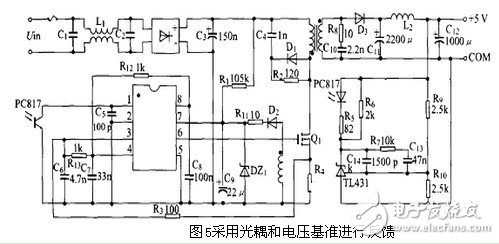4 Advantages of current mode control methods

The current mode control not only retains the output voltage feedback of the voltage type control, but also increases the inductor current feedback; and this current feedback acts as a ramp function of the PWM control converter, so that the sawtooth generator is no longer needed, and the performance of the system is obvious. The superiority. The characteristics of the current mode control method are as follows:

1. The system has fast input and output dynamic response and high stability;

2, high output voltage accuracy;

3. It has the inherent ability to control the power switch current;

4. Good parallel operation capability. Since the rate of change of the feedback inductor current is directly following the change of the input voltage and the output voltage. In the voltage feedback loop, the output of the error amplifier acts as a current reference signal, which directly controls the duty cycle of the power switch on and off compared with the feedback inductor current. Therefore, voltage feedback is an important issue in current-type power supply design. This article describes the design of the voltage feedback circuit when using the current-mode control chip uc3842.

5 Conclusion

Different feedback methods can be selected according to specific requirements. However, for the feedback circuit of multiple outputs, since the output precision is different for each output application, the ratio of each positive output terminal to the feedback amount is different in the feedback. According to the specific requirements, the design is specifically designed to meet the application requirements. For example, when the output of +5v +12v is required, the former is often used in high-precision situations, so the proportion in the feedback is relatively large, which is 60%. The latter is taken as 40%. Since there are multiple outputs, a superposition technique can be used in the secondary winding to reduce the number of transformer winding turns.

Super Silent Yangdong Diesel Generator

Silent Generator For Home Use,Super Silent Yangdong Diesel Generator,Silent Yangdong Generator,Silent Yangdong Diesel Generator

Jiangsu Lingyu Generator CO.,LTD , https://www.lygenset.com